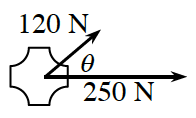### Home > PC3 > Chapter 9 > Lesson 9.2.1 > Problem9-107

9-107.Two forces with magnitudes of $120$ N and $250$ N act on an object of negligible mass as shown in the diagram at right.

1. Determine the magnitude and direction of the resultant force if $θ = 25^\circ$.

The resultant vector can be found by computing: $⟨120 \cos(25^\circ), 120\sin(25^\circ)⟩ + ⟨250 \cos(0^\circ), 250 \sin (0^\circ)⟩$

2. Determine the magnitude and direction of the resultant force if $θ = 45^\circ$.

3. Determine the magnitude and direction of the resultant force if $θ = 65^\circ$.

4. Express the magnitude of the resultant force as a function of $θ$, where $0º ≤ θ ≤ 180^\circ$.

The magnitude is the square root of the sum of each of the components squared.

$\sqrt{(120\cos(\theta)+250\cos(0^\circ))^2+(120\sin(\theta)+250\sin(0^\circ))^2}$